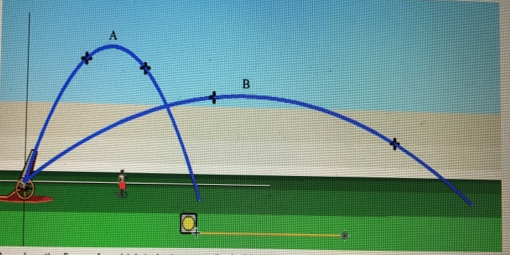# Problem: To understand how the trajectory of an object depends on its initial velocity, and to understand how air resistance affects the trajectory. For this problem, use the PhET simulation Projectile Motion. This simulation allows you to fire an object from a cannon, see its trajectory, and measure its range and hang time (the amount of time in the air). Click to launch video simulation Start the simulation. Press Fire to launch an object. You can choose the object by clicking on one of the objects in the scroll-down menu at top right (a cannonball is not among the choices). To adjust the cannon barrel’s angle, click and drag on it or type in a numerical value (in degrees). You can also adjust the speed, mass, and diameter of the object by typing in values. Clicking Air Resistance displays settings for (1) the drag coefficient and (2) the altitude (which controls the air density). For this tutorial, we will use an altitude of zero (sea level) and let the drag coefficient be automatically set when the object is chosen. Play around with the simulation. When you are done, click Erase and select a baseball prior to beginning Part A. Leave Air Resistance unchecked. The figure shows two trajectories, made by two balls launched with different angles and possibly different initial speeds. Based on the figure, for which trajectory was the ball in the air for the greatest amount of time?

###### FREE Expert Solution

For symmetrical launch:

Flight time:

$\overline{){\mathbf{t}}{\mathbf{=}}\frac{\mathbf{2}{\mathbf{v}}_{\mathbf{0}}\mathbf{s}\mathbf{i}\mathbf{n}\mathbf{\theta }}{\mathbf{g}}}$

The elevation/launch height is the same for both trajectories. The elevation of 1.2 m is small enough to allow us to conveniently analyze the situation by considering a symmetrical launch.

79% (2 ratings)###### Problem Details

To understand how the trajectory of an object depends on its initial velocity, and to understand how air resistance affects the trajectory. For this problem, use the PhET simulation Projectile Motion. This simulation allows you to fire an object from a cannon, see its trajectory, and measure its range and hang time (the amount of time in the air). Click to launch video simulation Start the simulation. Press Fire to launch an object. You can choose the object by clicking on one of the objects in the scroll-down menu at top right (a cannonball is not among the choices). To adjust the cannon barrel’s angle, click and drag on it or type in a numerical value (in degrees). You can also adjust the speed, mass, and diameter of the object by typing in values. Clicking Air Resistance displays settings for (1) the drag coefficient and (2) the altitude (which controls the air density). For this tutorial, we will use an altitude of zero (sea level) and let the drag coefficient be automatically set when the object is chosen. Play around with the simulation. When you are done, click Erase and select a baseball prior to beginning Part A. Leave Air Resistance unchecked.

The figure shows two trajectories, made by two balls launched with different angles and possibly different initial speeds. Based on the figure, for which trajectory was the ball in the air for the greatest amount of time?Frequently Asked Questions

What scientific concept do you need to know in order to solve this problem?

Our tutors have indicated that to solve this problem you will need to apply the Projectile Motion: Positive Launch concept. If you need more Projectile Motion: Positive Launch practice, you can also practice Projectile Motion: Positive Launch practice problems.